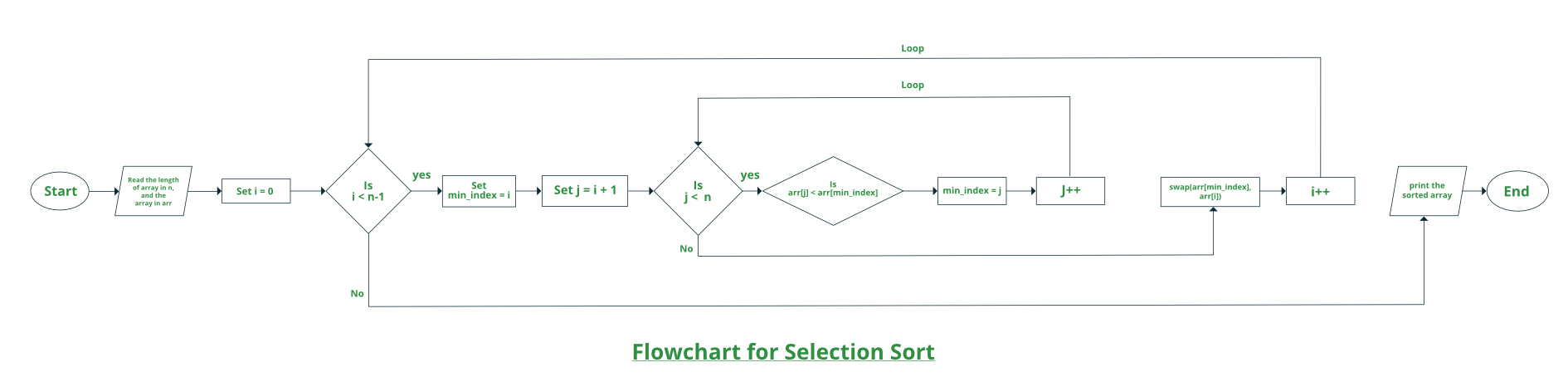GeeksforGeeks App
Open AppBrowser
Continue

# C++ Program For Selection Sort

The selection sort algorithm sorts an array by repeatedly finding the minimum element (considering ascending order) from unsorted part and putting it at the beginning. The algorithm maintains two subarrays in a given array.

• The subarray which is already sorted.
• Remaining subarray which is unsorted.

In every iteration of selection sort, the minimum element (considering ascending order) from the unsorted subarray is picked and moved to the sorted subarray.

###How selection sort works?

Lets consider the following array as an example: arr[] = {64, 25, 12, 22, 11}

First pass:

• For the first position in the sorted array, the whole array is traversed from index 0 to 4 sequentially. The first position where 64 is stored presently, after traversing whole array it is clear that 11 is the lowest value.
• Thus, replace 64 with 11. After one iteration 11, which happens to be the least value in the array, tends to appear in the first position of the sorted list.

Second Pass:

• For the second position, where 25 is present, again traverse the rest of the array in a sequential manner.
• After traversing, we found that 12 is the second lowest value in the array and it should appear at the second place in the array, thus swap these values.

Third Pass:

• Now, for third place, where 25 is present again traverse the rest of the array and find the third least value present in the array.
• While traversing, 22 came out to be the third least value and it should appear at the third place in the array, thus swap 22 with element present at third position.

Fourth pass:

• Similarly, for fourth position traverse the rest of the array and find the fourth least element in the array
• As 25 is the 4th lowest value hence, it will place at the fourth position.

Fifth Pass:

• At last the largest value present in the array automatically get placed at the last position in the array
• The resulted array is the sorted array.

### Approach:

• Initialize minimum value(min_idx) to location 0
• Traverse the array to find the minimum element in the array
• While traversing if any element smaller than min_idx is found then swap both the values.
• Then, increment min_idx to point to next element
• Repeat until array is sorted

Below is the implementation of the above approach:

## C++

 `// C++ program for implementation of ``// selection sort ``#include ``using` `namespace` `std;`` ` `//Swap function``void` `swap(``int` `*xp, ``int` `*yp) ``{ ``    ``int` `temp = *xp; ``    ``*xp = *yp; ``    ``*yp = temp; ``} `` ` `void` `selectionSort(``int` `arr[], ``int` `n) ``{ ``    ``int` `i, j, min_idx; `` ` `    ``// One by one move boundary of ``    ``// unsorted subarray ``    ``for` `(i = 0; i < n-1; i++) ``    ``{ ``       ` `        ``// Find the minimum element in ``        ``// unsorted array ``        ``min_idx = i; ``        ``for` `(j = i+1; j < n; j++) ``        ``if` `(arr[j] < arr[min_idx]) ``            ``min_idx = j; `` ` `        ``// Swap the found minimum element ``        ``// with the first element ``        ``swap(&arr[min_idx], &arr[i]); ``    ``} ``} `` ` `//Function to print an array``void` `printArray(``int` `arr[], ``int` `size) ``{ ``    ``int` `i; ``    ``for` `(i=0; i < size; i++) ``        ``cout << arr[i] << ``" "``; ``    ``cout << endl; ``} `` ` `// Driver program to test above functions ``int` `main() ``{ ``    ``int` `arr[] = {64, 25, 12, 22, 11}; ``    ``int` `n = ``sizeof``(arr)/``sizeof``(arr); ``    ``selectionSort(arr, n); ``    ``cout << ``"Sorted array: "``; ``    ``printArray(arr, n); ``    ``return` `0; ``} `

Output

```Sorted array:
11 12 22 25 64 ```

### Complexity Analysis of Selection Sort:

Time Complexity: The time complexity of Selection Sort is O(N2) as there are two nested loops:

• One loop to select an element of Array one by one = O(N)
• Another loop to compare that element with every other Array element = O(N)

Therefore overall complexity = O(N)*O(N) = O(N*N) = O(N2)

Auxiliary Space: O(1) as the only extra memory used is for temporary variable while swapping two values in Array. The good thing about selection sort is it never makes more than O(n) swaps and can be useful when memory write is a costly operation.

### Is Selection Sort Algorithm stable?

Stability : The default implementation is not stable. However it can be made stable. Please see stable selection sort for details.

### Is Selection Sort Algorithm in-place?

Yes, it does not require extra space.

My Personal Notes arrow_drop_up